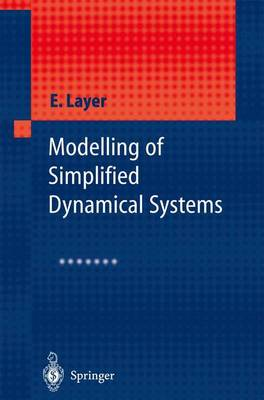•# Modelling of Simplified Dynamical Systems (Paperback)

(author)
£79.99
Paperback 171 Pages / Published: 05/11/2012
• We can order this

Usually dispatched within 3 weeks

Problems involving synthesis of mathematical models of various physical systems, making use of these models in practice and verifying them qualitatively has - come an especially important area of research since more and more physical - periments are being replaced by computer simulations. Such simulations should make it possible to carry out a comprehensive analysis of the various properties of the system being modelled. Most importantly its dynamic properties can be - dressed in a situation where this would be difficult or even impossible to achieve through a direct physical experiment. To carry out a simulation of a real, phy- cally existing system it is necessary to have its mathematical description; the s- tem being described mathematically by equations, which include certain variables, their derivatives and integrals. If a single independent variable is sufficient in - der to describe the system, then derivatives and integrals with respect to only that variable will appear in the equations. Differentiation of the equation allows the integrals to be eliminated and produces an equation which includes derivatives with respect to only one independent variable i. e. an ordinary differential equation. In practice, most physical systems can be described with sufficient accuracy by linear differential equations with time invariant coefficients. Chapter 2 is devoted to the description of models by such equations, with time as the independent va- able.

Publisher: Springer-Verlag Berlin and Heidelberg GmbH & Co. KG
ISBN: 9783642628566
Number of pages: 171
Weight: 284 g
Dimensions: 235 x 155 x 9 mm
Edition: Softcover reprint of the original 1st ed. 200